# Team:Hong Kong HKUST/Model Alkane mfcHielo by TEMPLATED## Alkane Metabolism Model

ASS enzymatic reaction model
Formation of alkylsuccinate is the first step of alkane metabolism. The enzyme involved was reported as alkylsuccinate synthase.

The overall enzymatic reaction: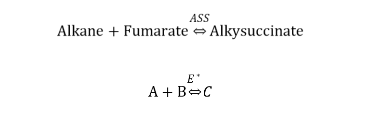The mechanism of this enzymatic reaction includes three main steps, H-abstraction, fumarate addition and thiyl radical regeneration . This model used n-butane as the modeled chemical and we assumed the general n-alkane will follow similar reaction mechanism. Each reaction was treated as a sub-mechanism consisting of 3 steps, reactant complex (RC) formation, transition state (TS) crossing and product separation. The following are the rate constants involved in the sub-mechanism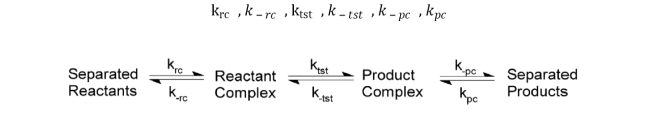The H-abstraction step (1) are modelled as,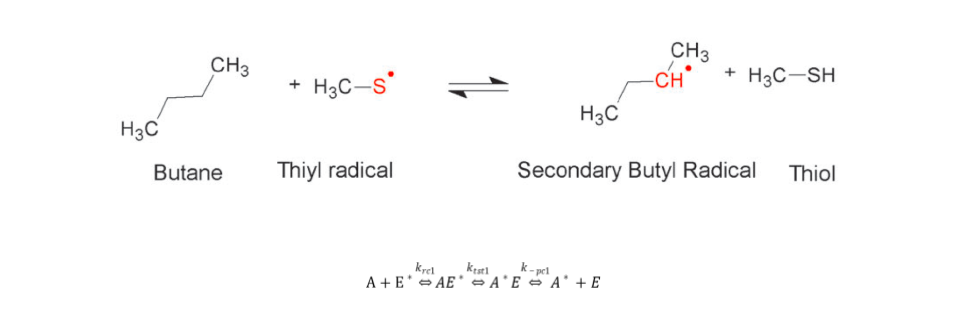The fumarate addition step (2) are modelled as,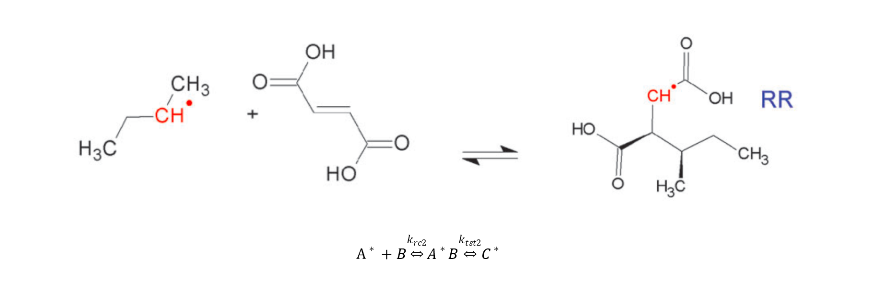The thiyl radical regeneration step (3) are modelled as,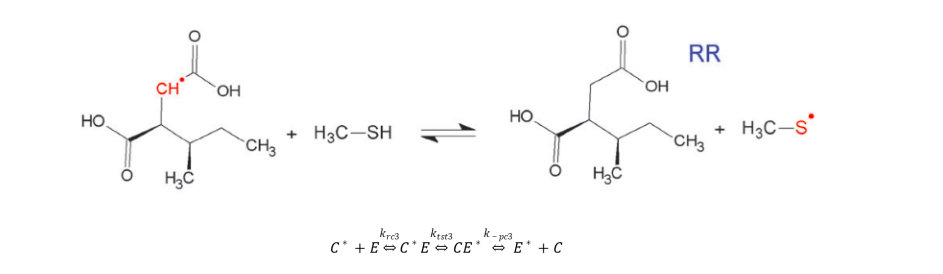Assume

(1)   The system is in a closed homogeneous batch reactor with infinite capacity

(2)   The reaction proceeds until final alkane concentration is negligible

(3)   Each step of the mechanism follows the Law of mass action

The reaction rates of each component are modelled as a system of ODE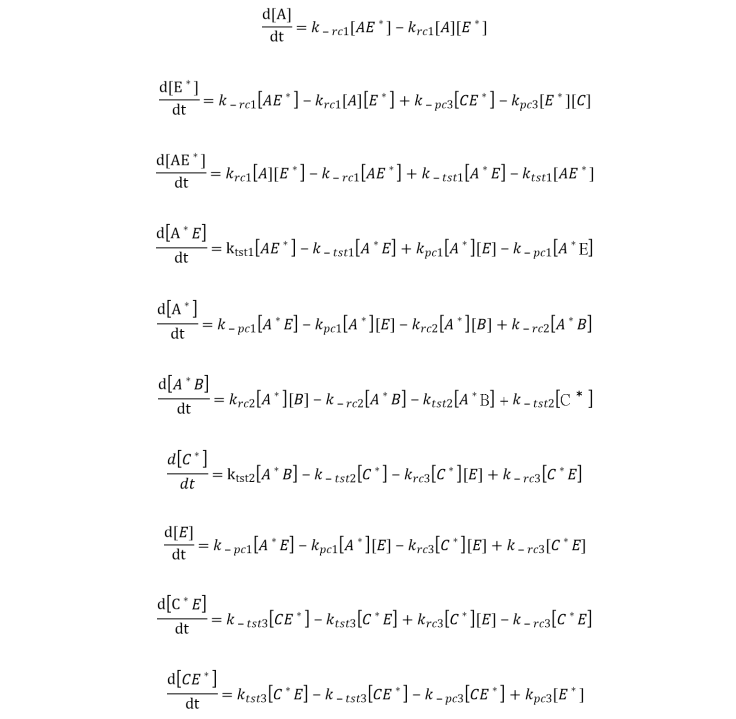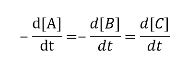Assume pseudo-steady state for the enzyme and intermediates, such that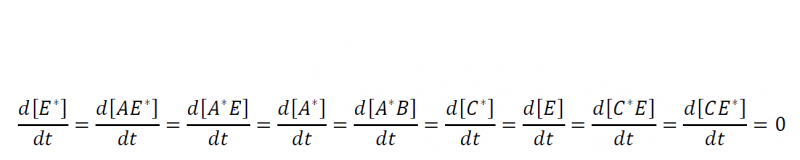Figure 3. Alkane degradation rate catalyzed by alkylsuccinate synthase

### Discussion

From this ASS enzymatic model, we obtained specific pseudo rate constant with respect to alkane equals to 2.48 X 10-5s-1
while we noticed that hexane reaction flux in shewanella is 6.04 X 10-7 mmol/gDW-h
from the FBA model.The slower prediction in the FBA model might due to limited transport rate of large alkane molecule into the cell.

Assume in 1 L batch reactor and general cell density for one unit of OD600 is 0.3 gDW/L, the hexane uptake rate is estimated to be by the following equation
Hexane uptake rate(mM/h) = hexane uptake flux(mmol/gDW-h) X Conversion Factor(GDW/L)

The ASS enzymatic reaction model predicts alkane conversion rate to reach 1.8 X 10-7 mM/h
when 0.02uM initial alkane concentration is supplied under excess fumarate condition. This provides insight that micro-molar level of alkane is possible to generate current up to 2.189 A/gDW

Initial alkane concentration required (mM) = Specific Rate Constant(h-1)/Alkane uptake rate(mMh-1)

(Please refer to this document for the list of parameters used.)

## REFERENCES:

1. A. Herath, B. Wawrik, Y. Qin, J. Zhou and A. Callaghan, "Transcriptional response of Desulfatibacillum alkenivoransAK-01 to growth on alkanes: insights from RT-qPCR and microarray analyses", FEMS Microbiology Ecology, vol. 92, no. 5, p. fiw062, 2016.
2. V. Bharadwaj, S. Vyas, S. Villano, C. Maupin and A. Dean, "Unravelling the impact of hydrocarbon structure on the fumarate addition mechanism – a gas-phase ab initio study", Physical Chemistry Chemical Physics, vol. 17, no. 6, pp. 4054-4066, 2015.